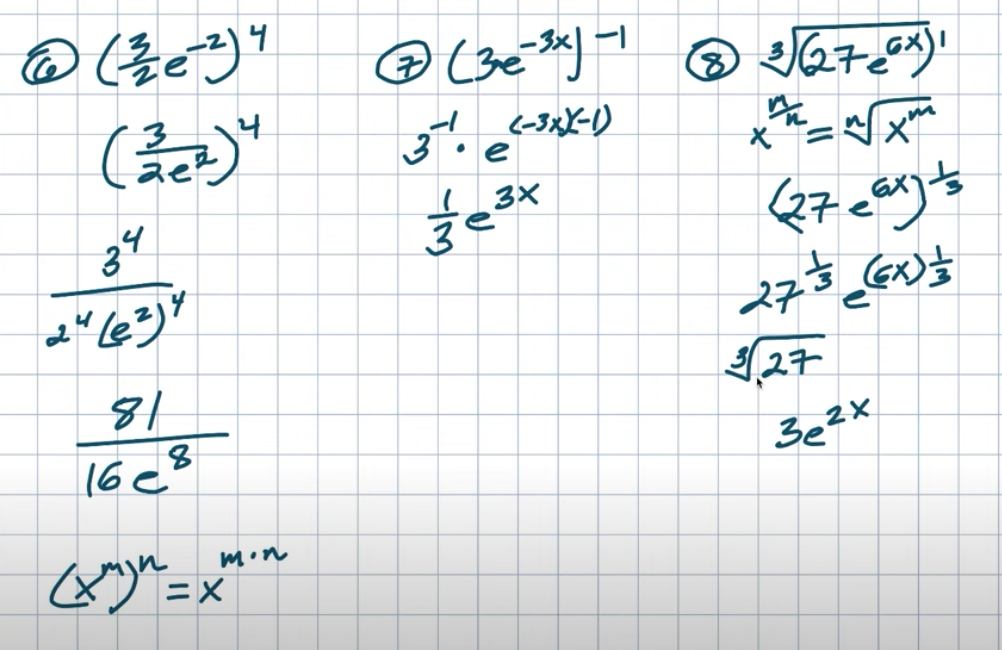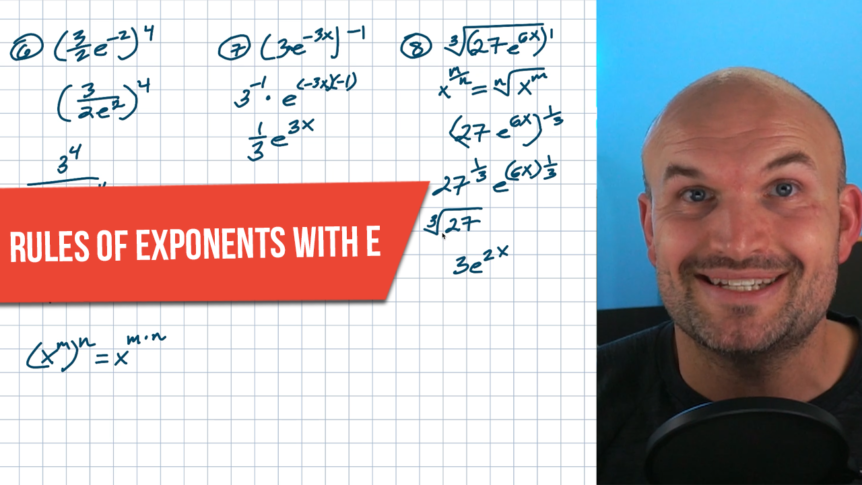# Apply Rules Of Exponents with e to Simplify

All right, so simplifying expressions with e using the rules of exponents can be tricky. There are 2 things I want you to know. One, e is a real number. Number 2, just treat e like any other variable.

Now you might be thinking, wait a minute, how is this going to help me out?

Well, see the problem is when students first encounter e, they treat it like some mysterious number that they no longer can do anything that they just learned in mathematics. And no, e is just like any other number. So just treat it like any other number. It’s not magically special were all of a sudden everything just got so much more confusing. E is a number, however, just like a number or a variable. When you’re simplifying expressions, the same properties that apply to variables and numbers are going to apply to e. So a lot of times I like to simplify expressions with X’s and Y’s and Ms and ends well. Again, treat those same properties with e.

So in this video, we’re going to work through 10 different examples to help you simplify expressions with e.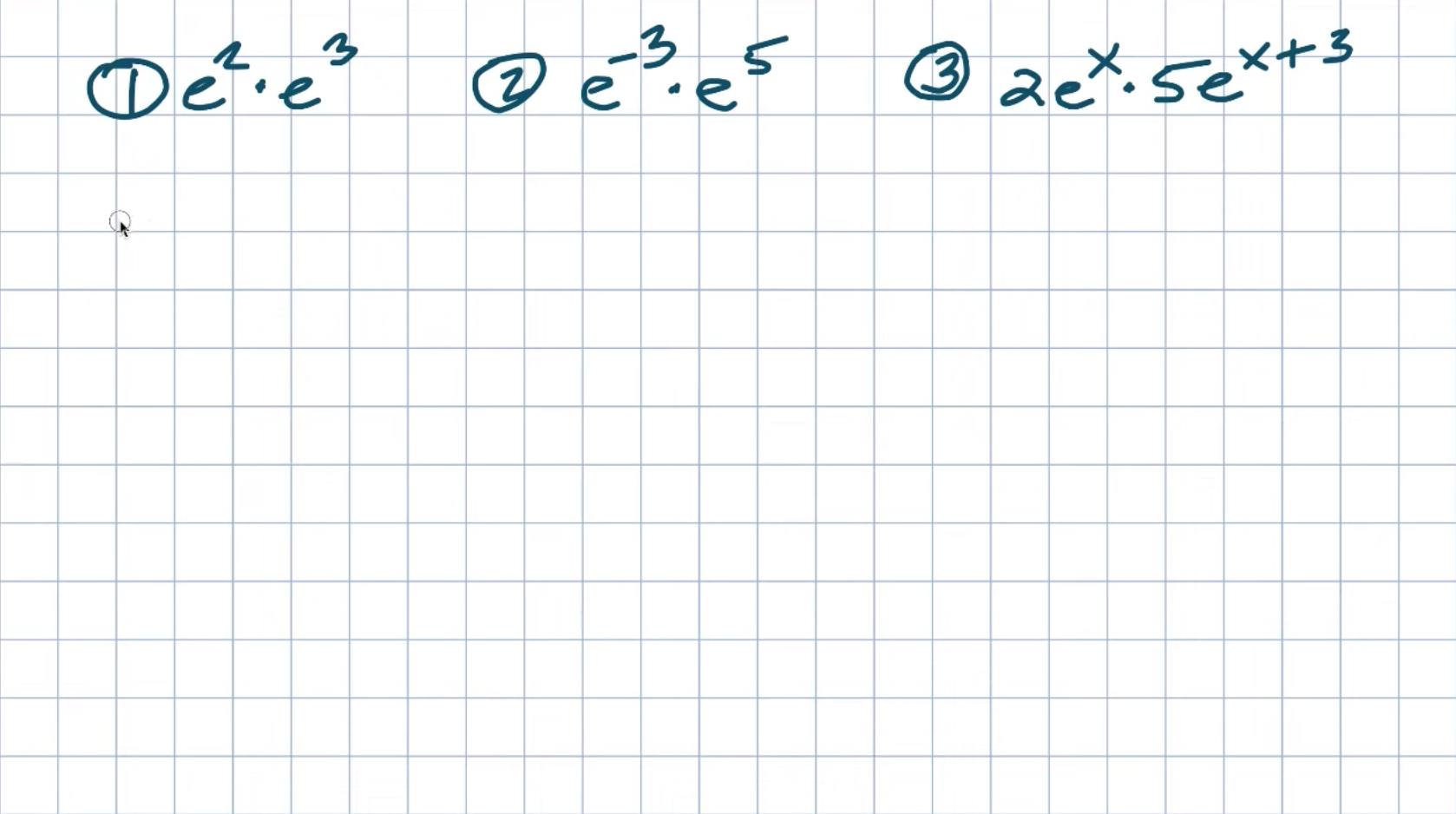Okay, in these first three examples, what you can see is I have an exponent multiplied by another exponent. The main thing I want you to think about is the product rule of exponents. Remember, if I had like X to the M times ax to the n, what do we do with the exponents? We just add the powers, right? And most students can kind of remember that. But again, it comes down to this idea of once we kind of get to like ease, it’s like they forget everything. So just to kind of go back to remembering treating this as like a variable, just go back and say, well, what if you had X squared times X cubed? Like, what would you do? You’d say, oh, that’s X to the 2 plus three, which is going to equal ax to the fifth. And yes, this is the exact same thing with ease. So don’t make e anything crazier than what it really is.

## Example #1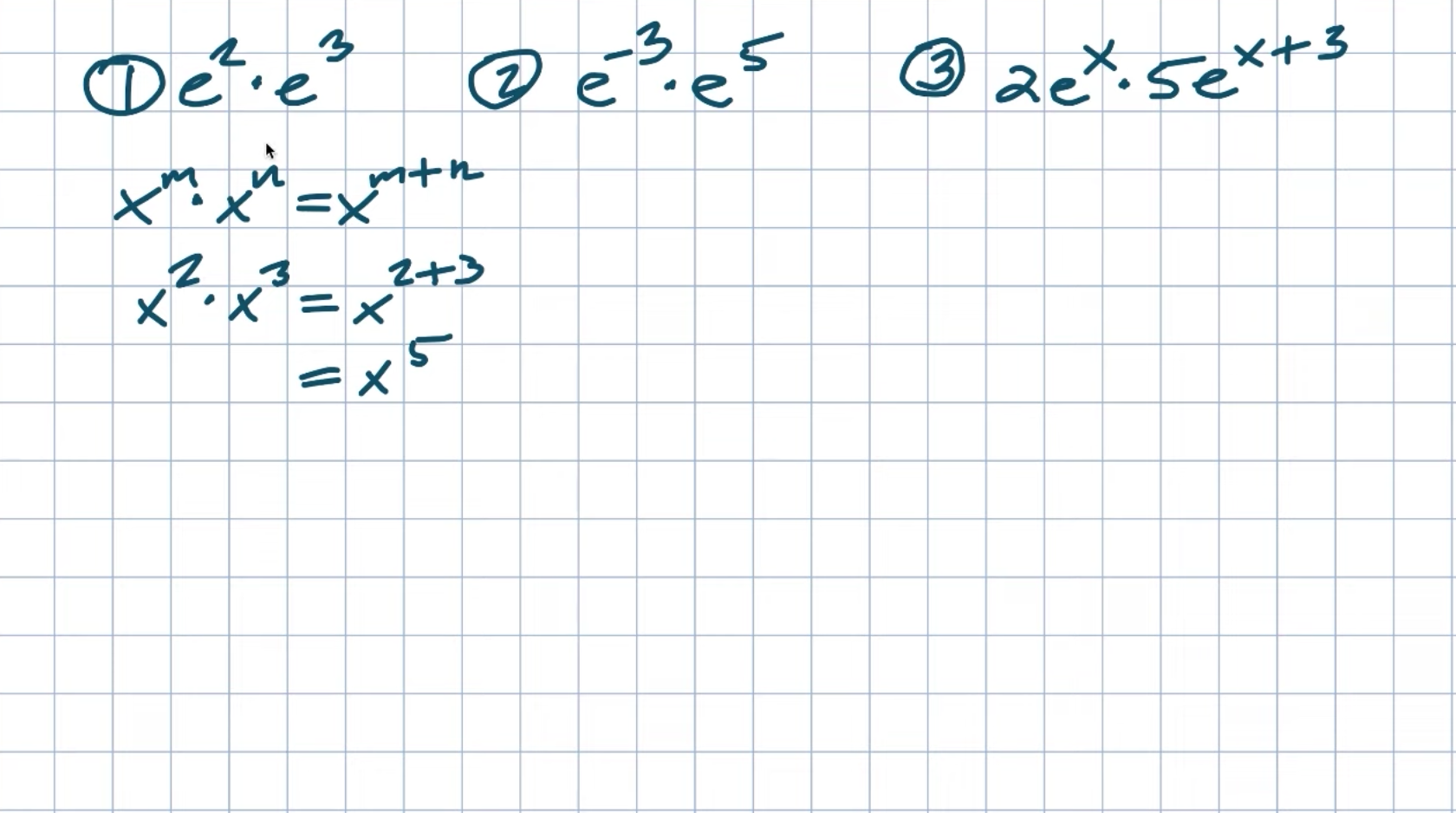If you’re going to simplify this, then e squared times e cubed is just going to be e to the 2 plus three, which is going to equal an e to the fifth power.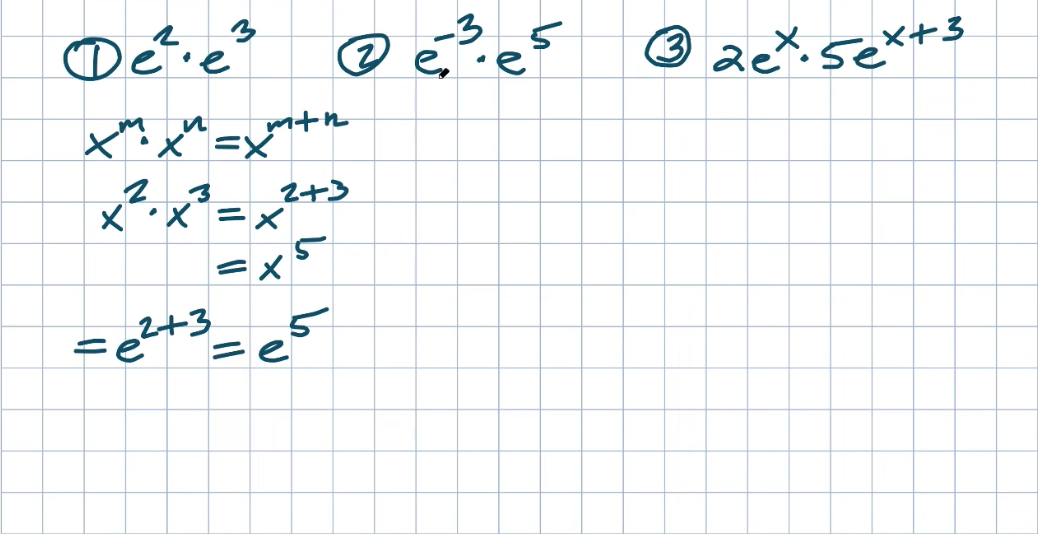## Example #2

So in the second example, we could definitely use the reciprocal identity member that any time we have an exponent raised to a negative power, we can go ahead and rewrite that as a positive power. So just remember, if I had like e to the negative M, I can rewrite that as a one-over ax to the M power. But again, in this example, I would just prefer to go ahead and use the product rule.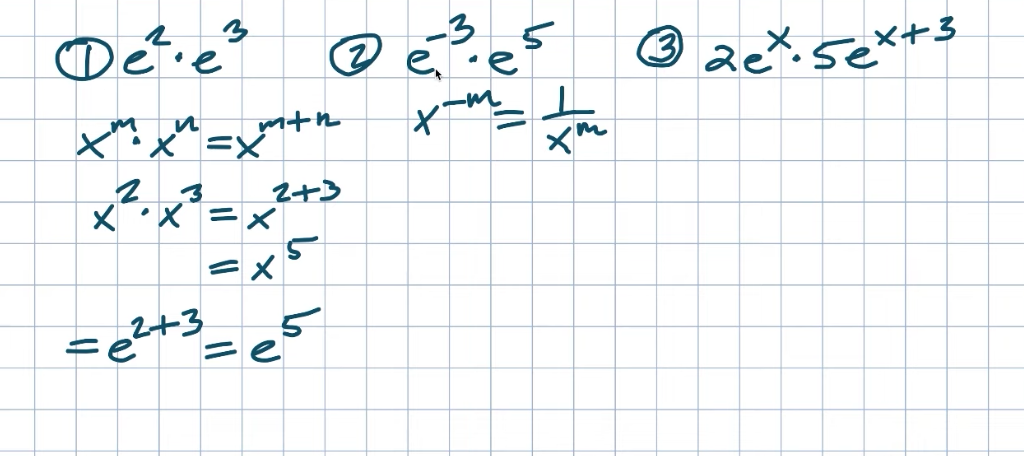So again, it doesn’t matter if I’m adding 2 positive powers or 2 negative powers or one negative and one positive. Again, remember, as long as the base is the same and you are applying multiplication, then just add the powers. So in this example, I have a negative three plus five, which is going to equal a positive 2. So therefore the answer is e to the second power.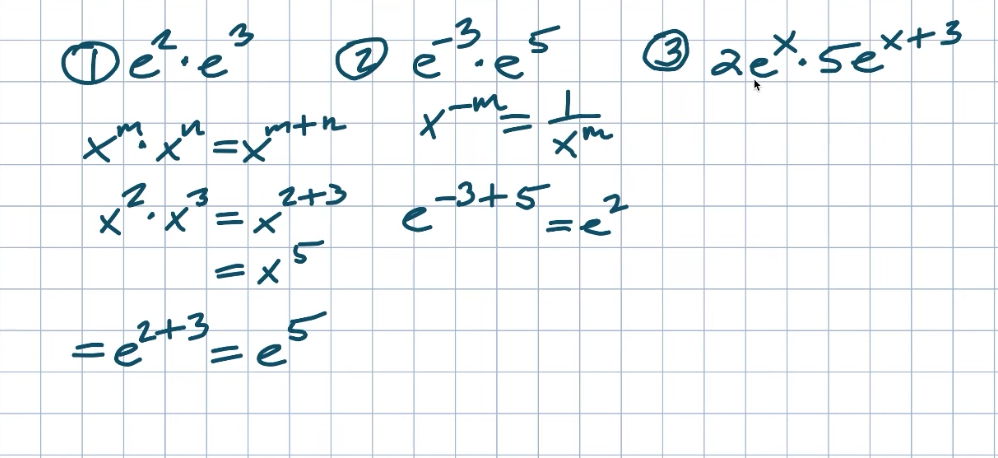## Example #3

Now in this example, we also have something that we call coefficients. Now, remember, that coefficients are not part of the rules of exponents. Just treat them as a second operation. We’re going to be applying multiplication with the exponents, and we’re going to be applying multiplication with the coefficients. So 2 times five is just going to be a 10 and e to the X times e to the X plus three. Again is going to be profitable we’re going to add the powers and we go ahead and add the powers. The only thing we can combine is the X plus X, which is going to be a 2 X. Therefore, in this example, we have a final answer of 10 times e to the 2 X plus three.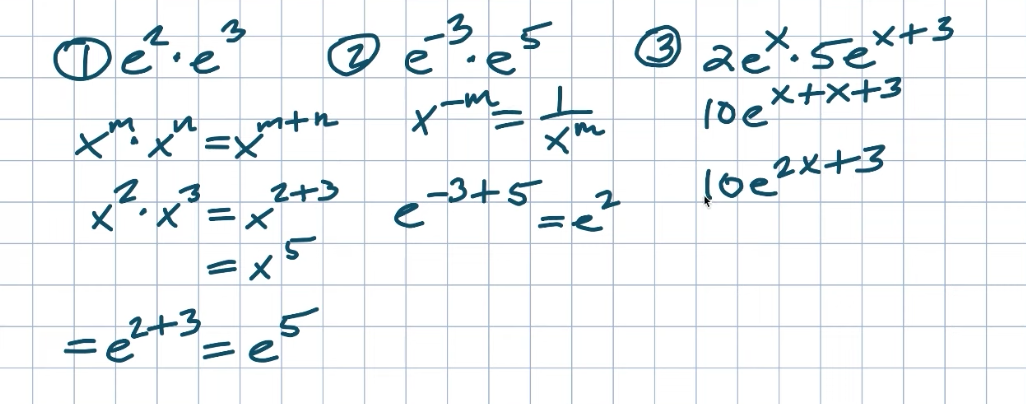## Example #4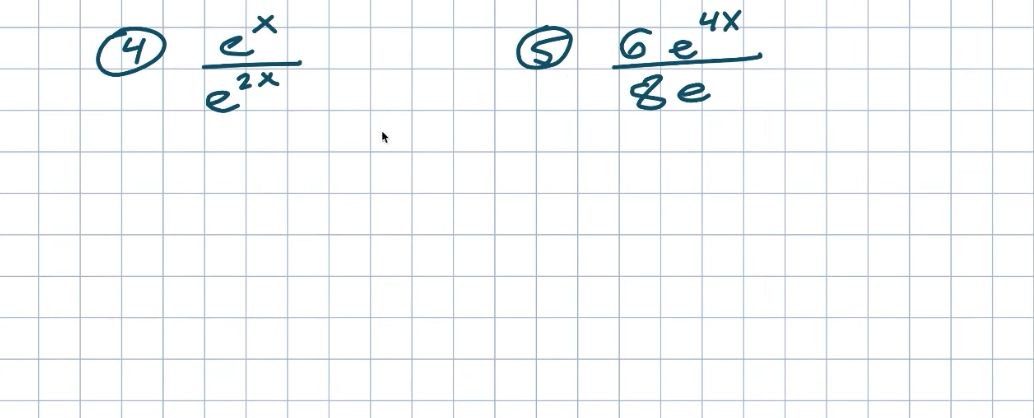Okay, in these 2 examples you can see we have a quotient, right? We’re applying for division. So the best thing we’re going to want to do in this case is to apply the quotient rule of exponents. And just remember, whenever we have an exponent like X to the M divided by X to then, we’re going to now subtract the power. So that’s going to be equivalent to X to M minus n. So in this example, I have e to the X divided by e to the 2 X. So therefore I can just go ahead and rewrite this as an e to the X minus 2 X. Now X minus 2 X is going to equal a e to the negative X. We can go back to our negative exponential and recognize that if I have e to the negative X and I want to rewrite that as a positive power, I can simply put that under one. So therefore my final answer, in this case, is with a positive power is going to be one over an e to the X.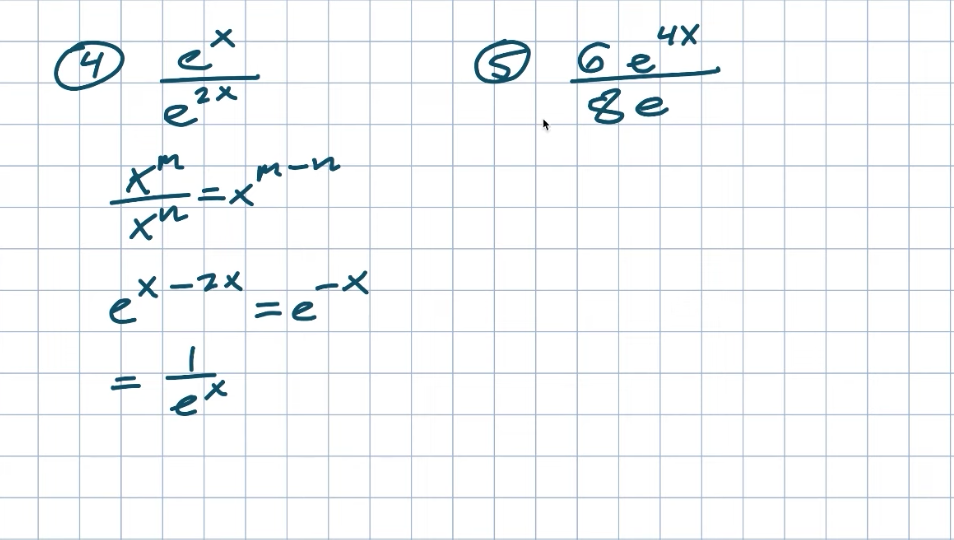## Example #5

Now in the second example again I have some coefficients, right? I have a six to the eight. So I’m going to treat that first because it’s like a separate operation from my rules of exponents. So now notice that eight does not evenly divide into six. So when this is the case, another thing we want to do is see if we can simplify it, see if there is a common number that six and eight share that we can divide evenly into both of them. And in this example, that common number is going to be 2. So to simplify the fraction six over eight, what I’m going to do is divide the six and eight both by that common number 2. And when I do that I get a six divided by 2, which is a three, and an eight divided by 2, which is going to be a four.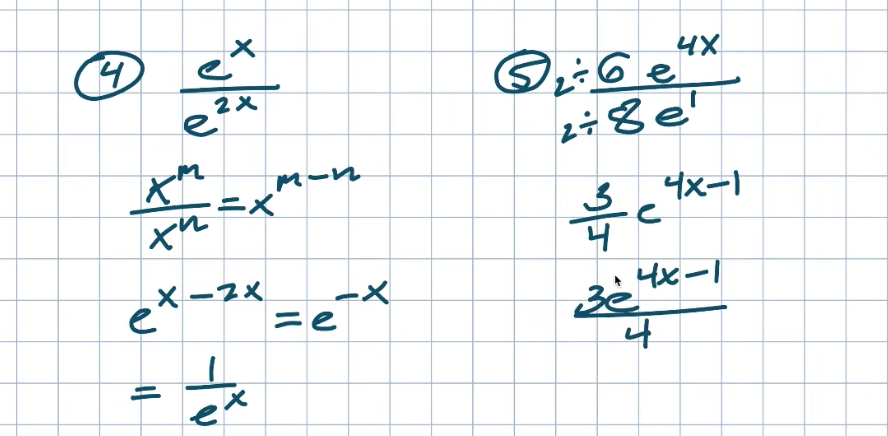Now that’s going to be multiplied by my exponent, which again, using the quotient rule, I’m going to subtract the powers. Now, some students will get confused here because E doesn’t have a power. Right? Well, again, there is a place value here that is going to be of one, not zero. Don’t make that mistake. I know you’re thinking it, but again, remember, anything raised to a zero power is going to equal one.

So e raised to the first power is just the same as e. But for the sake of applying our rules of exponents, we want to make sure we know that e is there. We just don’t want to have a four X here. So in this case, we’re going to have e to the four X minus one, and that’s going to be our final answer. Now, you could write it as a fraction multiplied times e, or we could rewrite this back as a rational problem with a three times e to the four X minus one, all divided by four. It really just kind of depends on what you or your teacher prefer or is required.

Now, in these three examples, you can see we have some extra steps, and that is perfectly okay. All we’re simply going to do is work on one property of excellence at a time.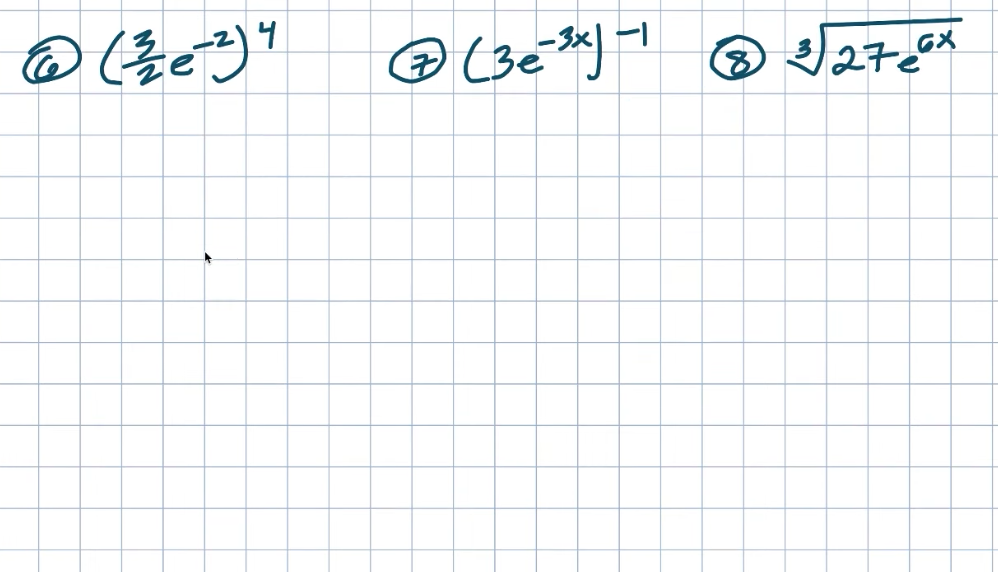## Example #6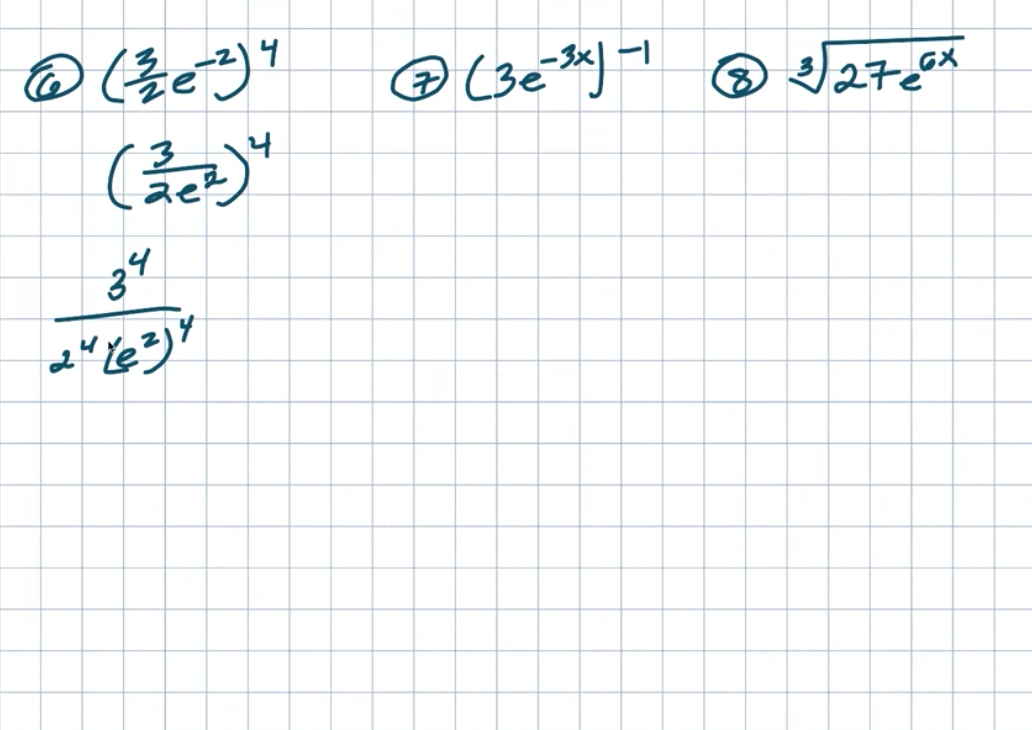Now, in this first example, what I would actually do is simplify inside my parentheses first. And typically when I mean simplify inside my parentheses, I mean get rid of negative power. So I’m going to rewrite this using the negative power rule as its reciprocal. And now you can see that I actually have a fraction being raised to a power. Now when we have that is going to be the case. What we can apply is what we call the power to quotient rule. What we can do is take each and every term and raise it to the fourth power. And again, this property works because each and every one of these terms is being separated by multiplication or division and take everything raised to the fourth power. Okay. And now that we distribute everything, now we just need to know what exactly is three to the fourth power, 2 to the fourth power, and e squared to the fourth power. So again, just remember, anything raised to the fourth power just means it’s being multiplied by itself four times like three times three times three times three. And that answer is going to be an 81. 2 to the fourth power is going to be a 16. And remember, when I have an exponent raised to another power, we need to use what we call the power role. So if I had X to the M raised to the N, we’re going to multiply our 2 powers. That’s going to be an X to the M times N. So 2 times four is just going to be 88. So this will be E to the 8th power. There’s nothing really I can simplify or reduce with 18 over 16. So that is going to be my final answer.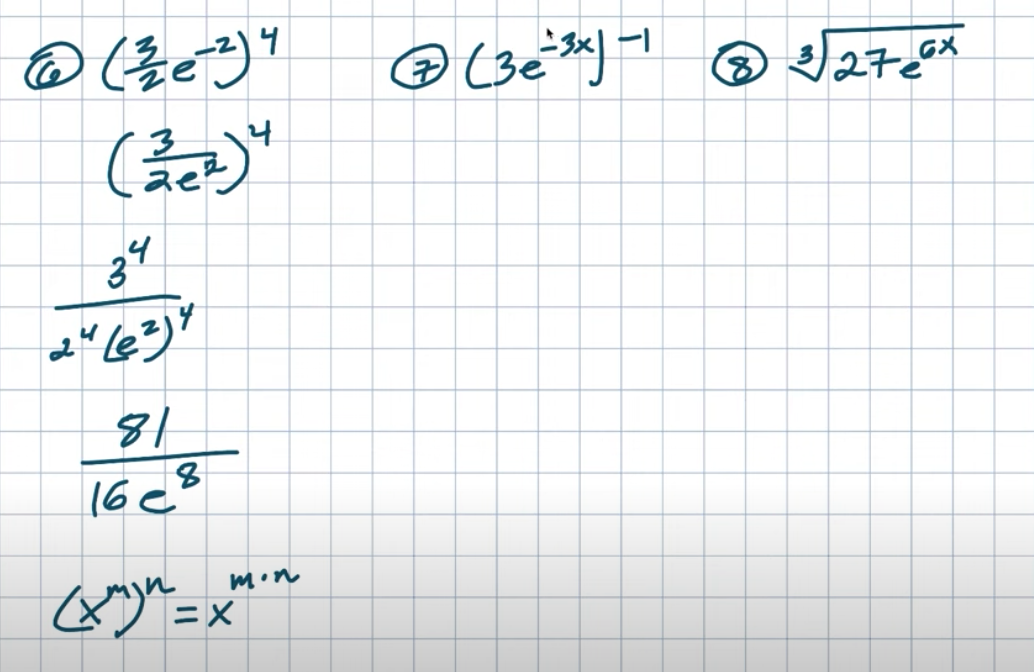Survive Math Class Checklist: Ten Steps to a Better Year: https://www.brianmclogan.com/email-ca🎯

## Example #7

Now in the second example, you can do the exact same thing. You can go ahead and rewrite this as it’s reciprocal to get a positive exponent. But then again, notice that I’m now going to be distributing that with a negative one. So all the extra work sometimes can just get compounded and make it a little bit more difficult. So sometimes when you see multiple negatives, it might just be easiest to apply the power to product rule first and then just go ahead and simplify afterward. So that’s exactly what I’m going to do here. I’m going to go ahead and distribute this negative one to both of my terms since they are separated by multiplication. And then I’ll go ahead and simplify. Okay. So I want you to pay attention to my use of parentheses. Notice how I have the three to the negative first power. Right. And then remember that’s times ae and I put parentheses around the negative three X and then times the negative because again, we’re not multiplying the negative times the negative three and the negative X or times an X. Right. Where it’s just that quantity negative three X is being multiplied by a negative one. And I also wanted to put parentheses around the negative one because I didn’t want it to look like a subtraction problem. Right. We’re dealing with multiplication. So when I go ahead and simplify this three to the negative first power, I can just rewrite that as a one-over three and then negative three X times negative one is just going to be a positive three X. And then you can leave this answer as a one three times e to the three X. Or you could write it as E to the three X divided by three. Again, whatever you kind of want.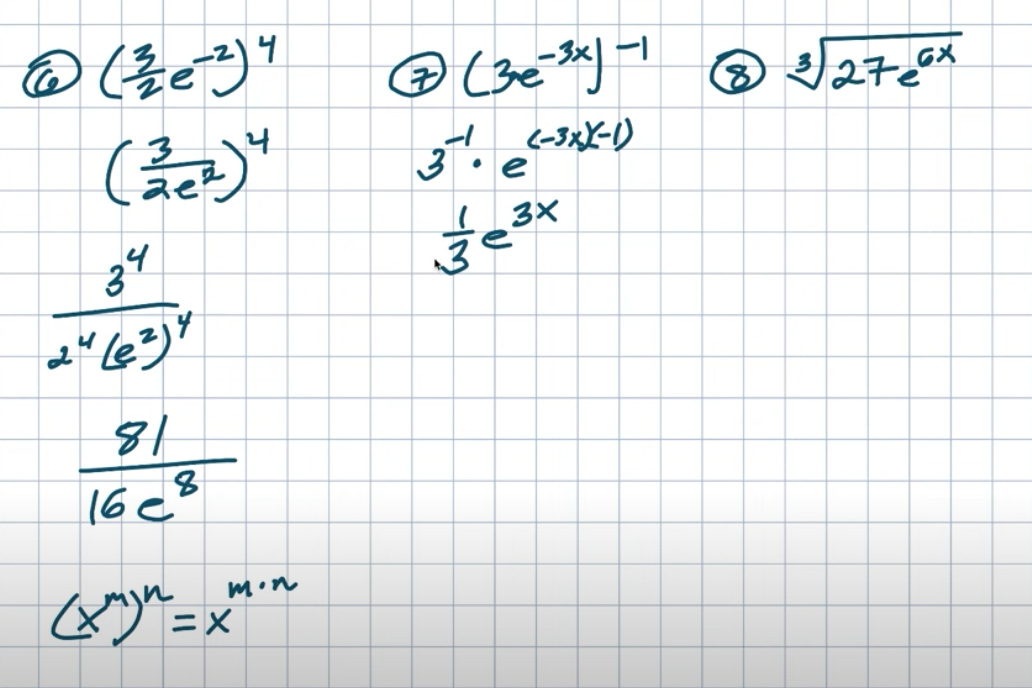## Example #8